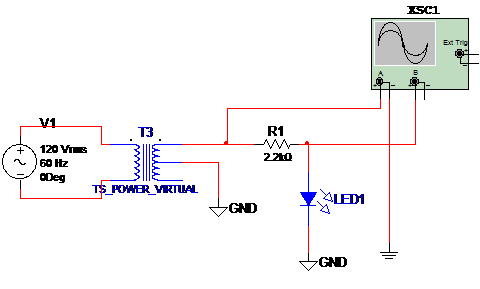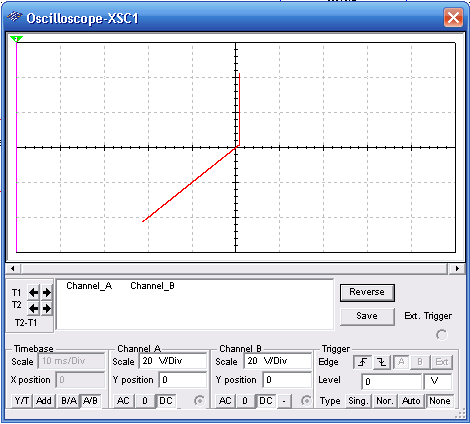## LED Test Circuit Using the Oscilloscope (MSB079E)

LEDs are diodes and can be tested with a simple configuration that allows the display of its characteristic curve in a oscilloscope. The circuit to make this simulation is given by figure 1. The signal source is a transformer powered from the AC power line. Figure 1 shows the circuit.Figure 1 – Circuit for LED test

The characteristic curve is observed in the virtual oscilloscope of the MultiSIM as given in figure 2. Observe the time base adjustment A/B.Figure 2 – Characteristic displayed by the oscilloscope